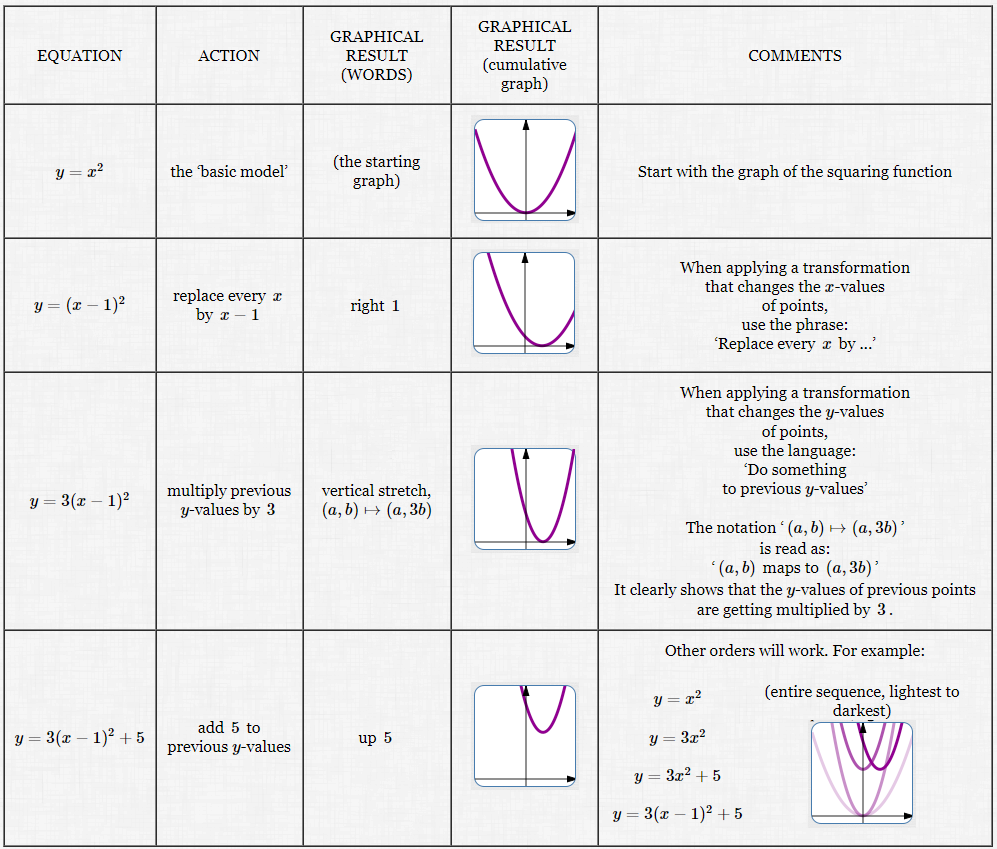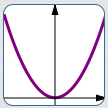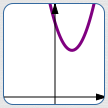﻿ Multi-Step Practice with all the Graphical Transformations

#Multi-Step Practice with all the Graphical Transformations

This lesson gives practice with all the graphical transformations:

Before practicing the multi-step exercises on this page, you should practice Single-step transformations, all mixed up. Jump to the exercises at the bottom, and click-click-click to check your understanding. When you're successfully answering all the question types, then you're ready for this current lesson!

## Example: Graphing Using Transformations

Graph $\,y = 3(x-2)^2 + 5\,$ by starting with a ‘basic model’ and successively applying graphical transformations.

(Here's the entire example in a single image! But it's too small to see easily—so keep reading ...Equation: $y = x^2$ Action: the ‘basic model’ Graphical Result (words): (the starting graph) Graphical Result (cumulative graph):Comments: Start with the graph of the squaring function
 Equation: $y = (x-1)^2$ Action: replace every $\,x\,$ by $\,x-1\,$ Graphical Result (words): right $\,1\,$ Graphical Result (cumulative graph):Comments: When applying a transformation that changes the $x$-values of points, use the phrase: ‘Replace every $\,x\,$ by ...’
 Equation: $y = 3(x-1)^2$ Action: multiply previous $y$-values by $\,3\,$ Graphical Result (words): vertical stretch, $\,(a,b) \mapsto (a,3b)\,$ Graphical Result (cumulative graph):Comments: When applying a transformation that changes the $y$-values of points, use the language: ‘Do something to previous $y$-values’ The notation ‘$\,(a,b) \mapsto (a,3b)\,$’ is read as: ‘$\,(a,b)\,$ maps to $\,(a,3b)\,$’ It clearly shows that the $y$-values of previous points are getting multiplied by $\,3\,.$
Equation: $y = 3(x-1)^2 + 5$
Action: add $\,5\,$ to previous $y$-values
Graphical Result (words): up $\,5\,$
Graphical Result
(cumulative graph):Other orders will work. For example:

 $y = x^2$ (entire sequence, lightest to darkest)$y = 3x^2$ $y = 3x^2 + 5$ $y = 3(x-1)^2 + 5$

## PRACTICE: Given the Desired Graphical Results, Produce the Equations

Give the Equations and Actions that produce the successive desired Graphical Results.